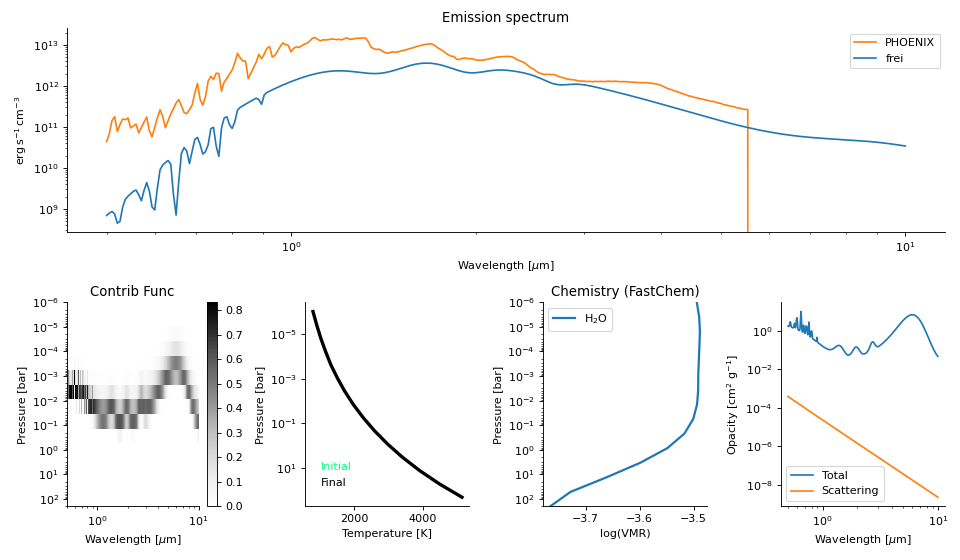# frei: Fast radiative transfer for exoplanet inference¶

Install the bleeding-edge version of frei with:

```python -m pip install git+https://github.com/bmorris3/frei
```

and go from zero to RT in five lines:

```import matplotlib.pyplot as plt
import astropy.units as u
from frei import Planet, Grid, load_example_opacity

# Define planetary system parameters
planet = Planet.from_hot_jupiter()

# Define a grid in wavelength, pressure, and temperature; set temperature
grid = Grid(
planet,
n_wl_bins=300,     # number of wavelength bins
n_layers=15,       # number of pressure layers
T_ref=2400 * u.K,  # reference temperature at 0.1 bar (~T_eff)
)

# Load synthetic opacities, for demonstration purposes only
);

# Compute emission spectrum
emission_result = grid.emission_spectrum(
# set n_timesteps>1 for iteration towards radiative equilibrium
n_timesteps=1
)

# Plot the "dashboard", showing important quantities
fig, ax = grid.emission_dashboard(*emission_result)
plt.show()
```